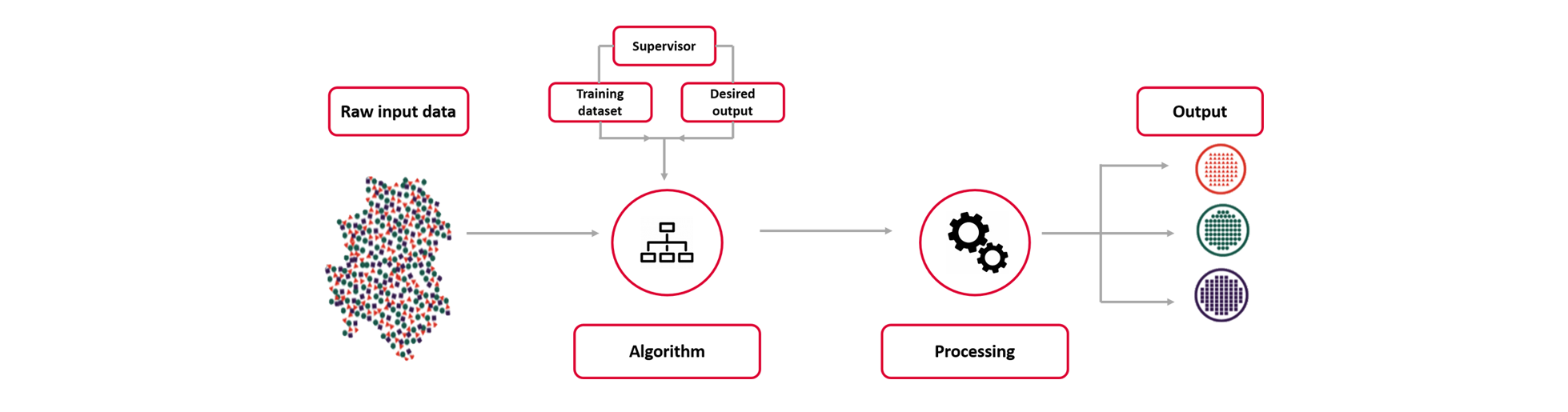# What is Supervised Machine Learning?

​​​​​​​​​​​​​​​​​​​​​​​​​​Nowadays, Machine Learning is hot and growing topic. It is a branch of Artificial Intelligence (AI), which uses data, algorithms, probabilities and statistics to allow computers to learn by themselves, without explicit computing. We can think of machine learning as teaching computers to learn. There are three categories of machine learning : supervised learning, unsupervised learning and reinforcement learning. In this article, we will be diving into what is known as supervised learning, but more specifically into the regression technique for supervised learning.​​​​​​​​​​​​​​​​​​​

### ​​​​​​​What is Supervised Learning?

Supervised learning is a predictive modelling technique, characterized by the making of an algorithm which learns to map an input to a particular output. This is achieved by training the model on a labeled dataset, which includes a set of input variables and their corresponding output variables. The training process is repeated until a satisfying performance is achieved. During each iteration, the machine creates a set of rules between the input and the output data. This process allows the model to learn from the dataset and apply the rules to accurately predict the output when a new input data is given.Supervised learning can be classified into two techniques: classification and regression.
Below, we will be focusing on the latter. For more information on regression models, classification and Linedata’s use of these techniques, keep an eye out for upcoming articles.

### Regression

​​​​​​​Unlike classification which predicts categories or labelling, regression models predict a continuous-valued output based on an independent input variable. This technique is used whenever the required output prediction is a continuous numerical variable, such as for Weather forecasting or Market trends. Different regression models exist and differ based on the relationship between the dependent and independent variables considered, as well as the number of independent variables being used in the model. Some of the main regression algorithms include simple and multiple linear regression, Poisson regression and Support Vector Regression (SVR).

Hence, regression analysis is a form of inferential statistics, used to find trends in our data. There exist multiple different regression algorithms with the common goal of determining a continuous-valued output based on an independent input variable.

### A use case of supervised machine learning​​​​​​​Furthermore, supervised machine learning algorithms are taking over many processes, in banking, which used to be manual. For instance, data scientists and engineers at Bank of America have created Predictive Intelligence Analytics Machine (PRIAM), an AI prediction system that uses a network of supervised machine learning algorithms. PRIAM allows to understand the relationship trends between equity capital market (ECM) deals and investors as well as to maximize the demand for ECM transactions by predicting the best investors for a deal based on the equity offering details, historical deal participation, trading and client touch point information, and market data.

For more details, please see: “Bank of America brings AI to equity capital markets”, CIO, March 2, 2020.

Hopefully this article will have given you background information on Supervised Machine Learning and it’s branch of regression models. In the following articles, we will dive into the main algorithms used in supervised regression learning and an example of a use case at Linedata.